# Cumulative Quiz #1 for Kaʻi

This quiz will test your knowledge of the kaʻi you have learned so far (up to Lessons 0608). These include ka, ke, kēlā, kēia, kēnā, he, and kekahi. If you need to, you can see the index page for the 0600 Series of lessons to go over the lessons up to this point.

1.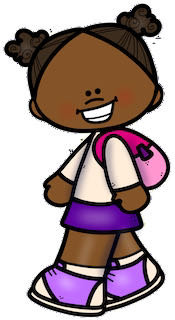the child
A.
B.
C.
D.
2.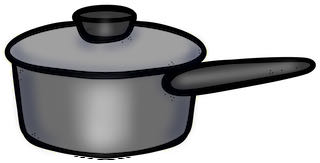another pot
A.
B.
C.
D.
3.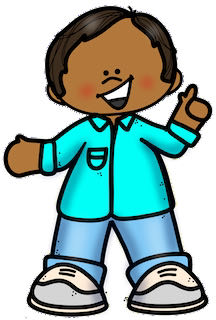the hand
A.
B.
C.
D.
4.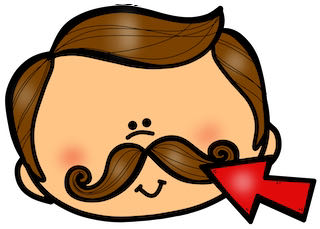that mustache (on the person you are talking to)
A.
B.
C.
D.
5. a pillow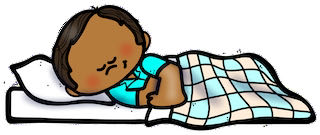a pillow
A.
B.
C.
D.
6.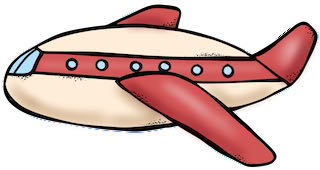that airplane (it's flying in the sky far away)
A.
B.
C.
D.
7.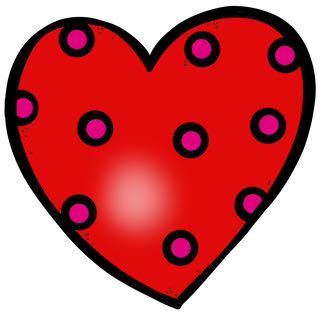that heart (imagine you are pointing away from yourself)
A.
B.
C.
D.
8.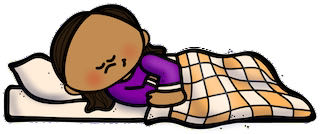the blanket
A.
B.
C.
D.
9.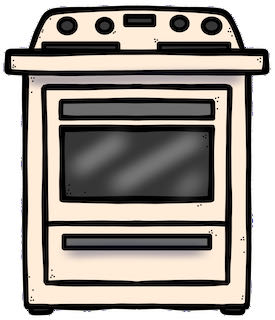the stove
A.
B.
C.
D.
10.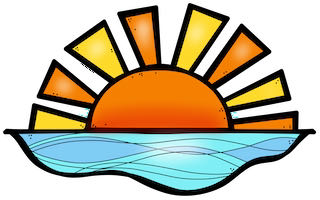another day
A.
B.
C.
D.
11.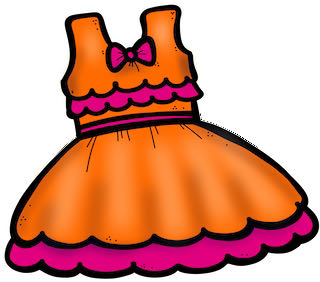a lady's dress
A.
B.
C.
D.
12.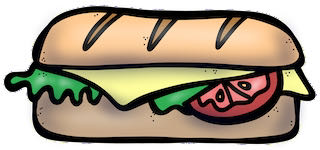the sandwich
A.
B.
C.
D.
13.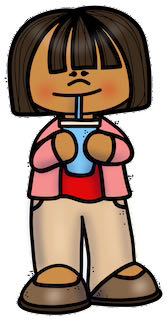a cup
A.
B.
C.
D.
14.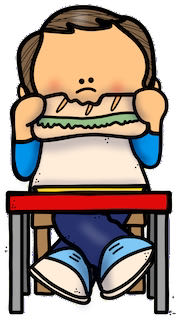the shoe
A.
B.
C.
D.
15.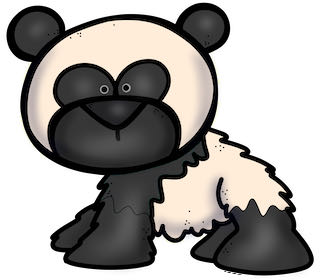another panda bear
A.
B.
C.
D.
16.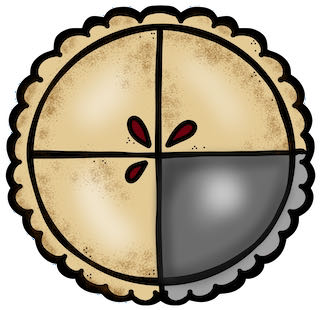another apple pie
A.
B.
C.
D.
17.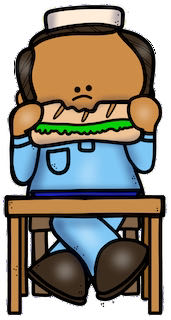this hat (imagine it's on your head)
A.
B.
C.
D.
18.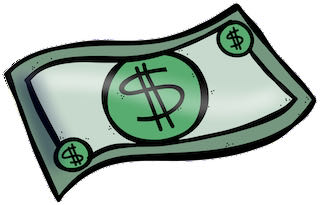this money (imagine you are holding it)
A.
B.
C.
D.
19.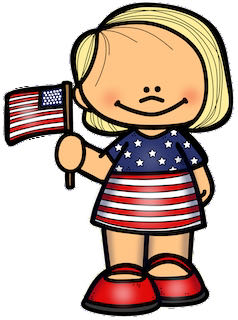this flag (imagine it's in your hand)
A.
B.
C.
D.
20.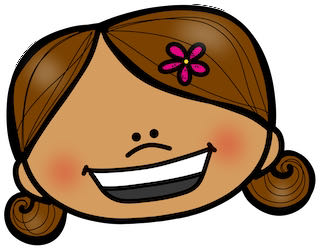that ribbon (close to the person you are talking to)
A.
B.
C.
D.